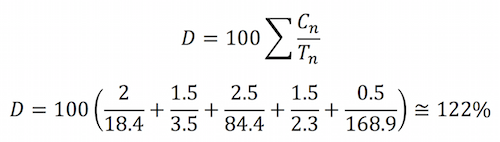# Calculating OSHA Employee Permissible Exposure Limit for NoiseIn 1970 Congress passed the Occupational Safety and Health Act. The act created The Occupational Safety and Health Administration (OSHA). It granted OSHA the authority to adopt existing federal standards or national consensus standards. In 1968 the American Conference of Government Industrial Hygienists published employee threshold limit values (TLVs) for hazardous substances. In 1971, per the act, OSHA adopted these standards calling them Employee Permissible Exposure Limits (PELs).

The PEL of a particular hazard constitutes the acceptable level that an employee can be exposed to during a given workday. For example, the PEL for asbestos exposure is 0.01 g/m3 for an eight-hour shift. An employee can be exposed to levels below that amount without personal protective equipment. If an employee’s asbestos exposure is at or above that level, his or her employer must provide them with adequate protection equipment: HEPA respirators, Tyvek suits, and amended water.

To determine if an employee has been exposed above the PEL, OSHA requires the contractor to monitor its employees and analyze the data by applying an eight-hour time weighted average calculation (TWA) to the collected data. In this article, we will explore OSHA’s time weighted average formula and demonstrate how to calculate an employee’s exposure to workplace place noises.

OSHA’s Table G-16 specifies the maximum number of hours per day a worker may be exposed to certain sound levels. An eight-hour exposure to 90 dBA is considered a 100% “dose”, the maximum allowable. Likewise, 6 hours at 92 dBA is also a 100% dose. Less-than-maximum times at a certain level would correspond to a less-than-maximum doses: 4 hours at 90 dBA would be a 50% dose.

## OSHA’s Table G-16

 Sound Level, slow response (dBA) Duration per day (hours) 85 16 90 8 92 6 95 4 97 3 100 2 102 1.5 105 1 110 0.5 115 0.25

Typically, workers may be exposed to different noise levels during the course of an eight-hour shift. To calculate the dosage in such scenarios, the partial times at the different sound levels would be added up. The following formula can be used to determine the percent dose for a day with different sound levels:D – The percentage dose
Cn – The actual hours at a certain sound level
Tn – The allowable time for that sound level (from Table G-16)

As an example, we can calculate the percentage dose for a worker who is exposed to 4 hours of 90 dBA and 4 hours of 85 dBA:This dose (75%) is acceptable since it is less than or equal to 100%.

For sound levels not listed on the chart, the following formula can be used to determine the allowable time T in hours:To determine the allowable exposure to 96 dBA, we would calculate as follows:## Time-Weighted Average (TWA)

TWA is another way to consider dose. The TWA is given in dBA, and shows the exposure as an 8-hour day at a constant sound level rather than as a percentage of the maximum level allowed. For example, a 50% dose is equivalent to a TWA of 85 dBA. To convert from percentage dose (D) to TWA, the following formula can be used:Which can be slightly simplified to:A dose of 79% would be equivalent to a time-weighted average of about 88.3 dBA. This is calculated as follows:## Putting the Formulas to Work

Let’s say that you work in a factory and throughout the day you deliver raw materials to different departments. Throughout the day you are exposed to a variety of noise levels. Let’s use the following noise levels to determine your overall exposure to noise during your workday:

• 2 hours at 84 dBA
• 1.5 hours at 96 dBA
• 2.5 hours at 73 dBA
• 1.5 hours at 99 dBA
• 0.5 hours at 68 dBA

First, we need to calculate the allowable times for an 8-hour day at these different sound levels. None of these dBA values is on the chart, so we can use the formula from above to calculate the allowable times:Doing the math will determine the following times (T), in hours:

 Sound Level, slow response (dBA) Duration per day (hours) 84 18.4 96 3.5 73 84.4 99 2.3 68 168.9

Now we need to sum these up using the formula:This dose is greater than 100%, so this is unacceptable and measures must be taken to reduce the worker’s exposure.

To calculate the equivalent sound level of this dose, we can calculate the TWA as follows:The above scenario is equivalent to the worker being exposed to 91.4 dBA over a full 8-hour workday.

## Noise DosimeterToday employees could be equipped with small wearable noise dosimeters. As the employee wears the dosimeter, the dosimeter records the noise level the employee is exposed to during the day. At the end of work shift, the dosimeter will calculate the TWA, minimum and maximum exposure levels and noise. At the end of the work shift, it can be plugged into computer where a statistical graph can be printed out. The dosimeter is worn on the employee’s shoulder. It must be calibrated in the field before use and periodically sent to an accredited lab for testing.

Related Articles:

Sound Waves and Their Characteristics Explained

Mayan Basketball & The Reflection of Sound Waves

Thermaxx Products:

Learn about Thermaxx Noise Insulation Jackets

This entry was posted in Machine Maintenance, Sound Insulation. Bookmark the permalink.